" 张三出国之后，变成了张思睿 "# Naïve Bayes Classifier Types

## Feature

Naive: assume the features are indenpendent, have little/no correlation with each other

Highly efficient.

## Type

Bernoulli: binary features(e.g. present/absent)

Multinomial: discrete features(e.g. count)

Gaussian: continuous/real-valued features

## Application

from sklearn.naive_bayes import GaussianNB

X_train, X_test, y_train, y_test = train_test_split(X_C2, y_C2, random_state=0)

nbclf = GaussianNB().fit(X_train, y_train)
plot_class_regions_for_classifier(nbclf, X_train, y_train, X_test, y_test,
'Gaussian Naive Bayes classifier: Dataset 1')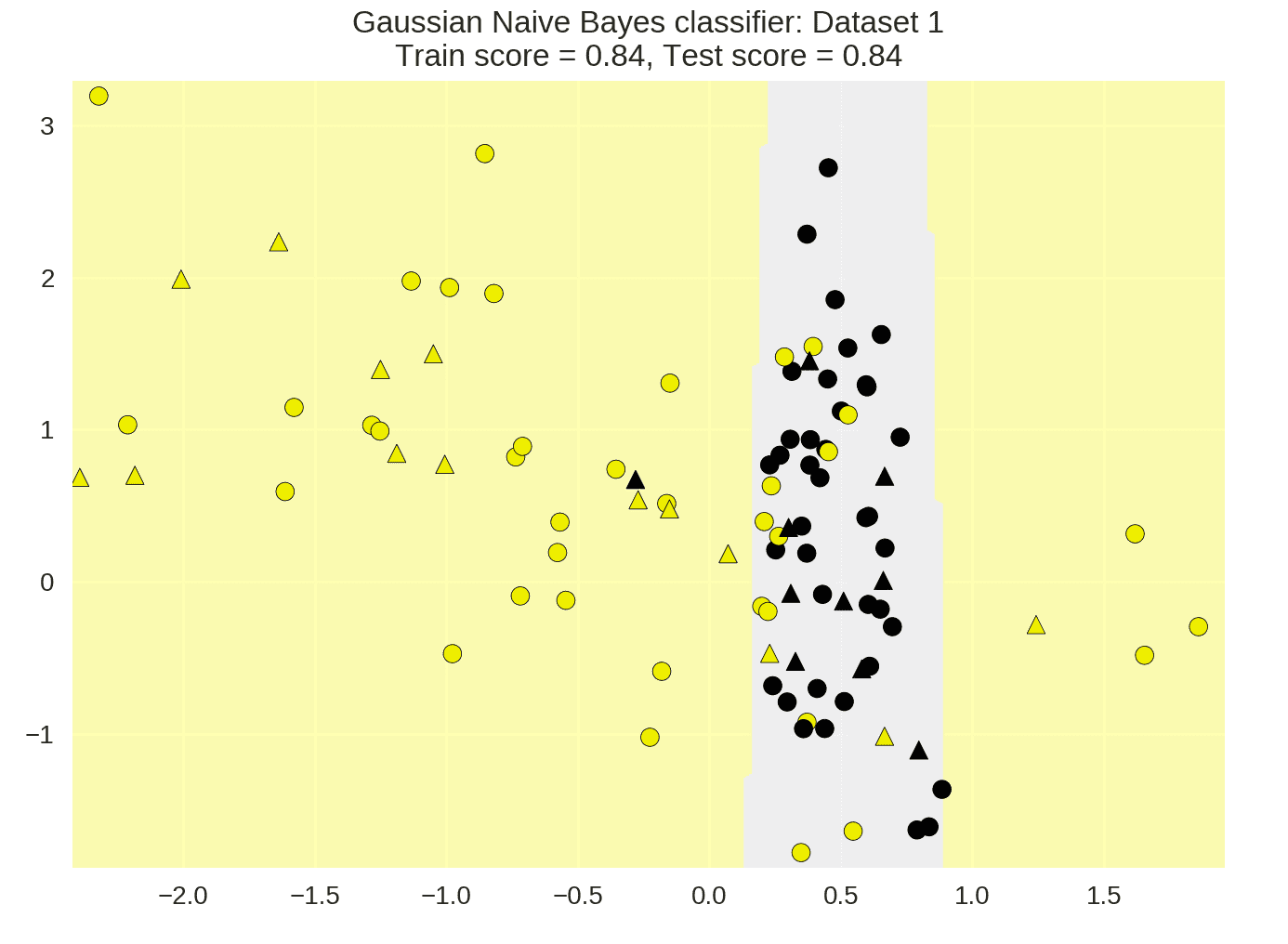X_train, X_test, y_train, y_test = train_test_split(X_D2, y_D2,
random_state=0)

nbclf = GaussianNB().fit(X_train, y_train)
plot_class_regions_for_classifier(nbclf, X_train, y_train, X_test, y_test,
'Gaussian Naive Bayes classifier: Dataset 2')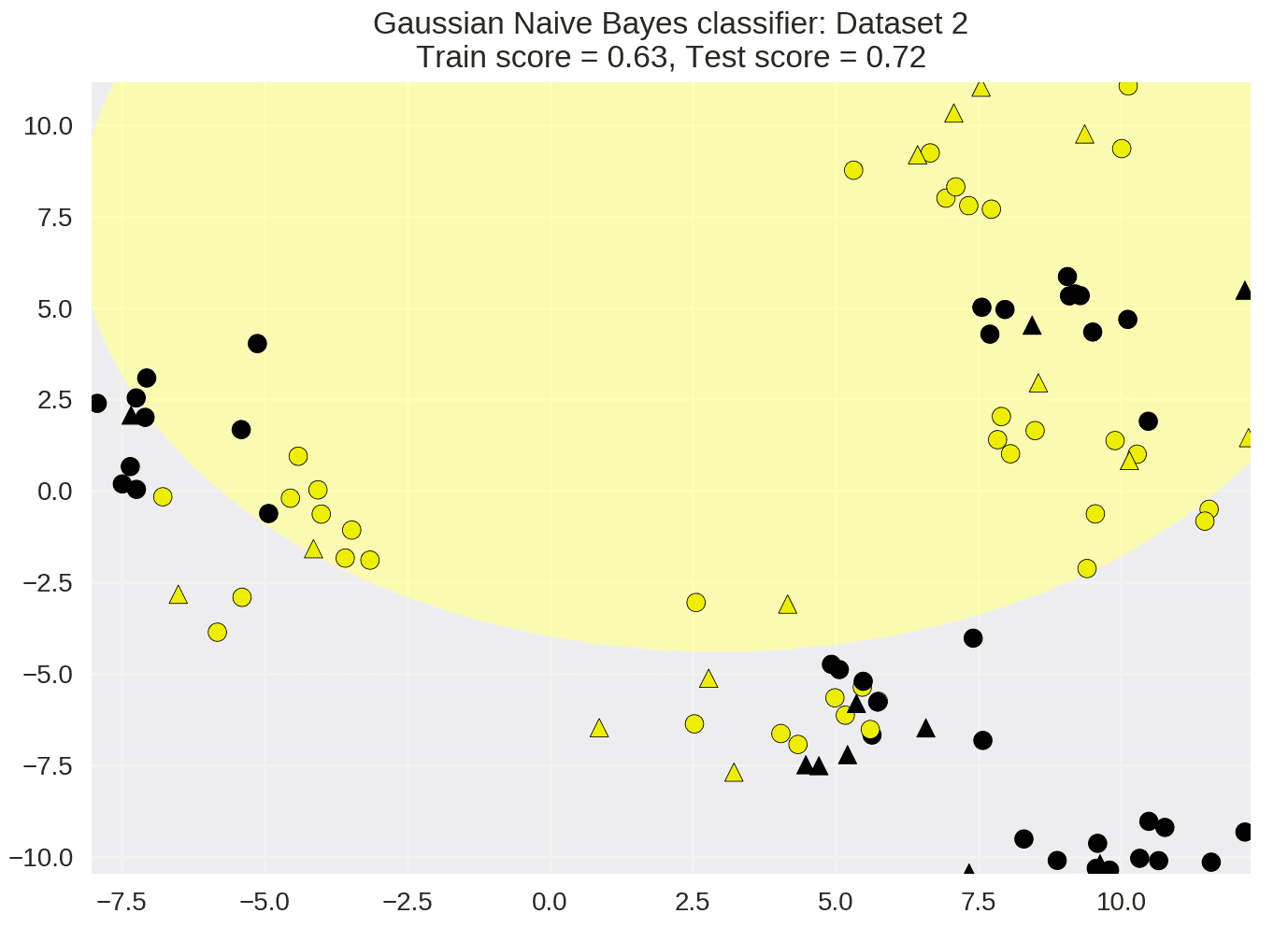# Decision Tree

### Feature

• An ensemble of trees
• Widely used
• sklearn module:
• Classification: RandomForestClassifier
• Regression: RandomForestRegressor
• Many decision trees have better generalization than one decision trees(might overfitting)

### Random Forest Process

boost strip samples

max_feature=1: forests with diverse and more complex trees

max_features=<close to number of features>: similar forest with simpler trees

regression:

• mean of individual tree predictions

classification:

• Each tree gives probability for each class
• Probabilities averaged across trees
• Predict the class with highest probability

n_estimators: number of trees(default10)

max_depth: depth of each tree(default none)

n_jobs: number of cores to use in parallel during training

from sklearn.ensemble import RandomForestClassifier
from sklearn.model_selection import train_test_split

X_train, X_test, y_train, y_test = train_test_split(X_D2, y_D2,
random_state = 0)
fig, subaxes = plt.subplots(1, 1, figsize=(6, 6))

clf = RandomForestClassifier().fit(X_train, y_train)
title = 'Random Forest Classifier, complex binary dataset, default settings'
plot_class_regions_for_classifier_subplot(clf, X_train, y_train, X_test,
y_test, title, subaxes)

plt.show()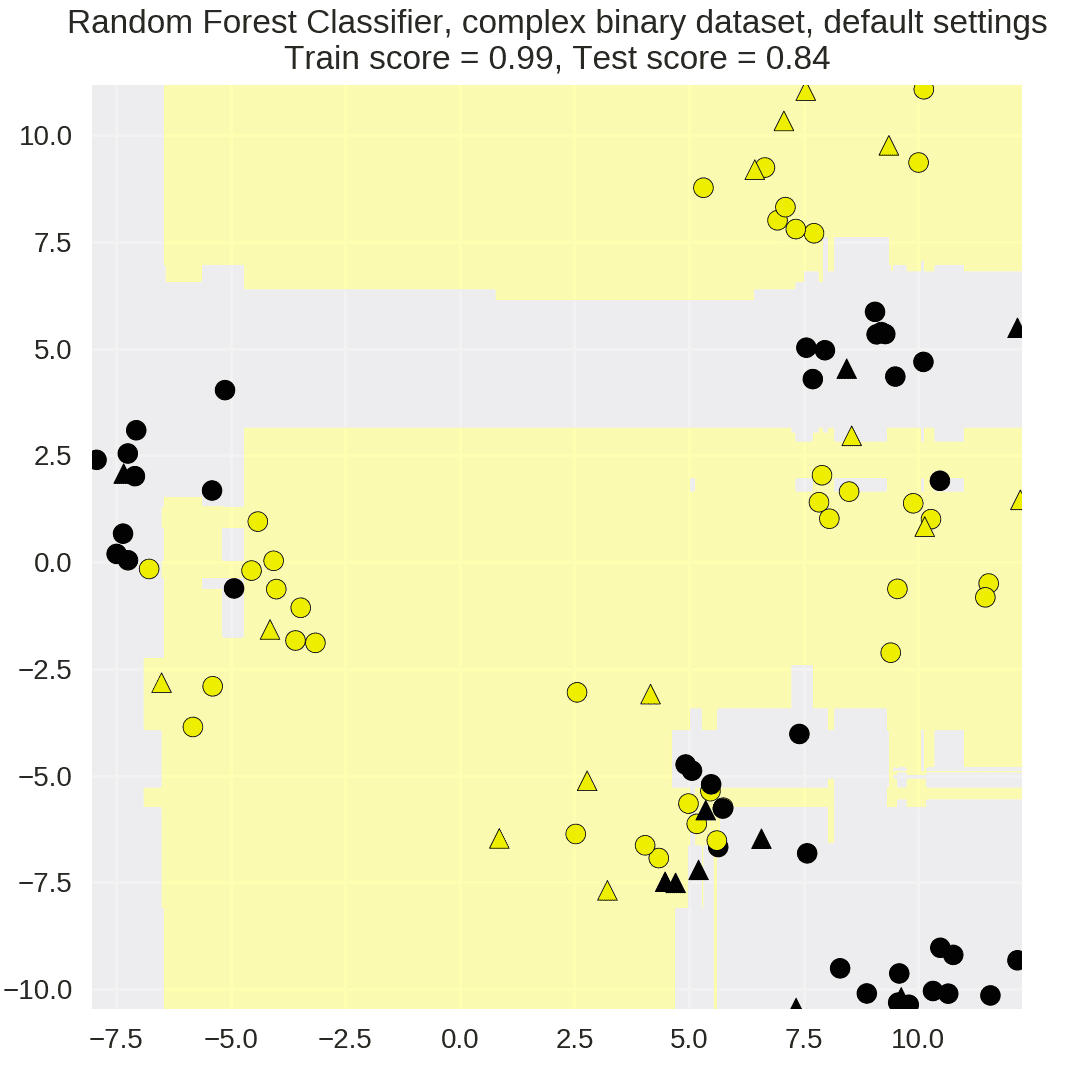The key idea of gradient boosted decision trees is that they build a series of trees.

n_estimators: set # of small decision trees to use

learning_rate: controls emphasis on fixing errors from previous iteration

max_depth: control depth of

from sklearn.ensemble import GradientBoostingClassifier
from sklearn.model_selection import train_test_split

X_train, X_test, y_train, y_test = train_test_split(X_D2, y_D2, random_state = 0)
fig, subaxes = plt.subplots(1, 1, figsize=(6, 6))

title = 'GBDT, complex binary dataset, default settings'
plot_class_regions_for_classifier_subplot(clf, X_train, y_train, X_test,
y_test, title, subaxes)

plt.show()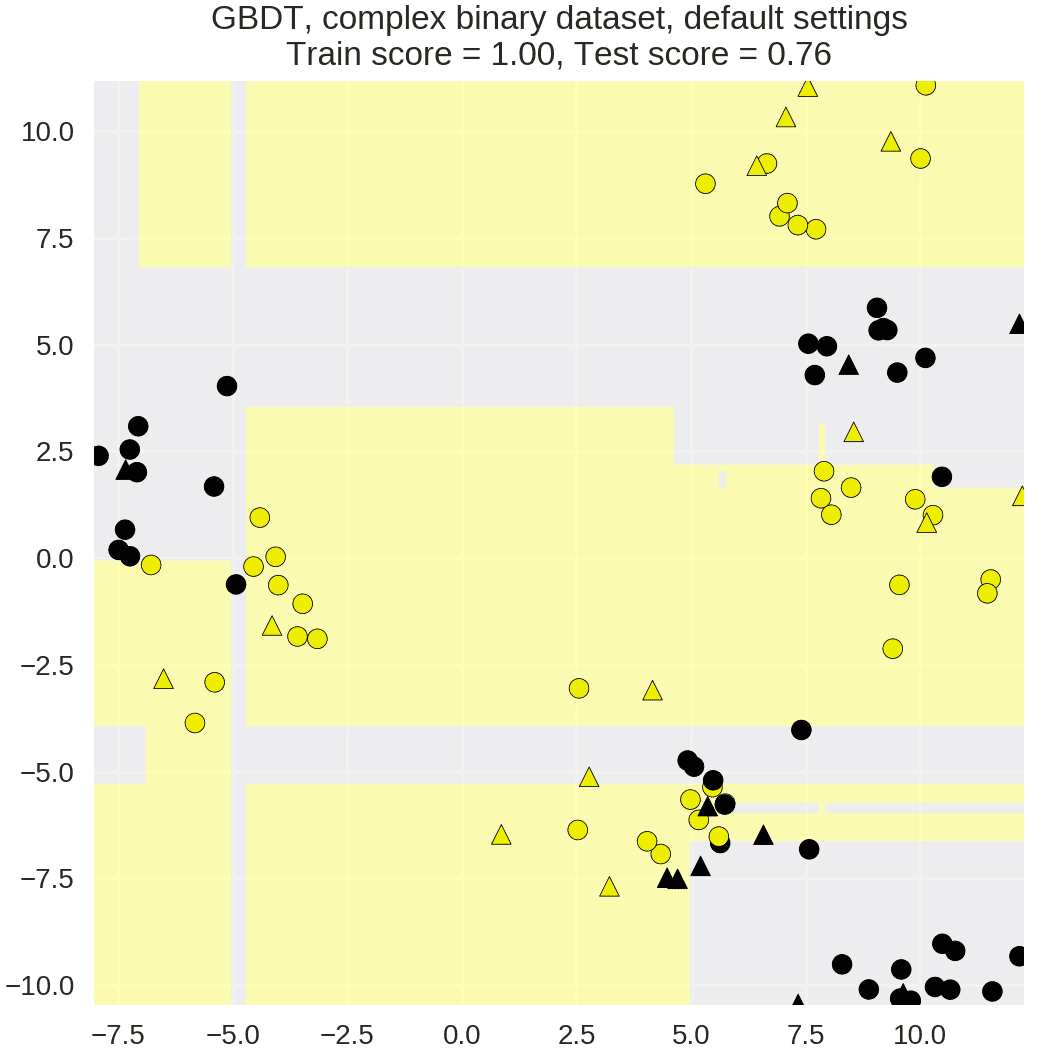# Neural Networks

Details of Neural Networks

## Intro

multi-layer perceptron with N hidden layers

Activation Functions:

1. Relu (also called 'Rectified Linear Unit' via Wikipedia):

1. tanh:
1. logistic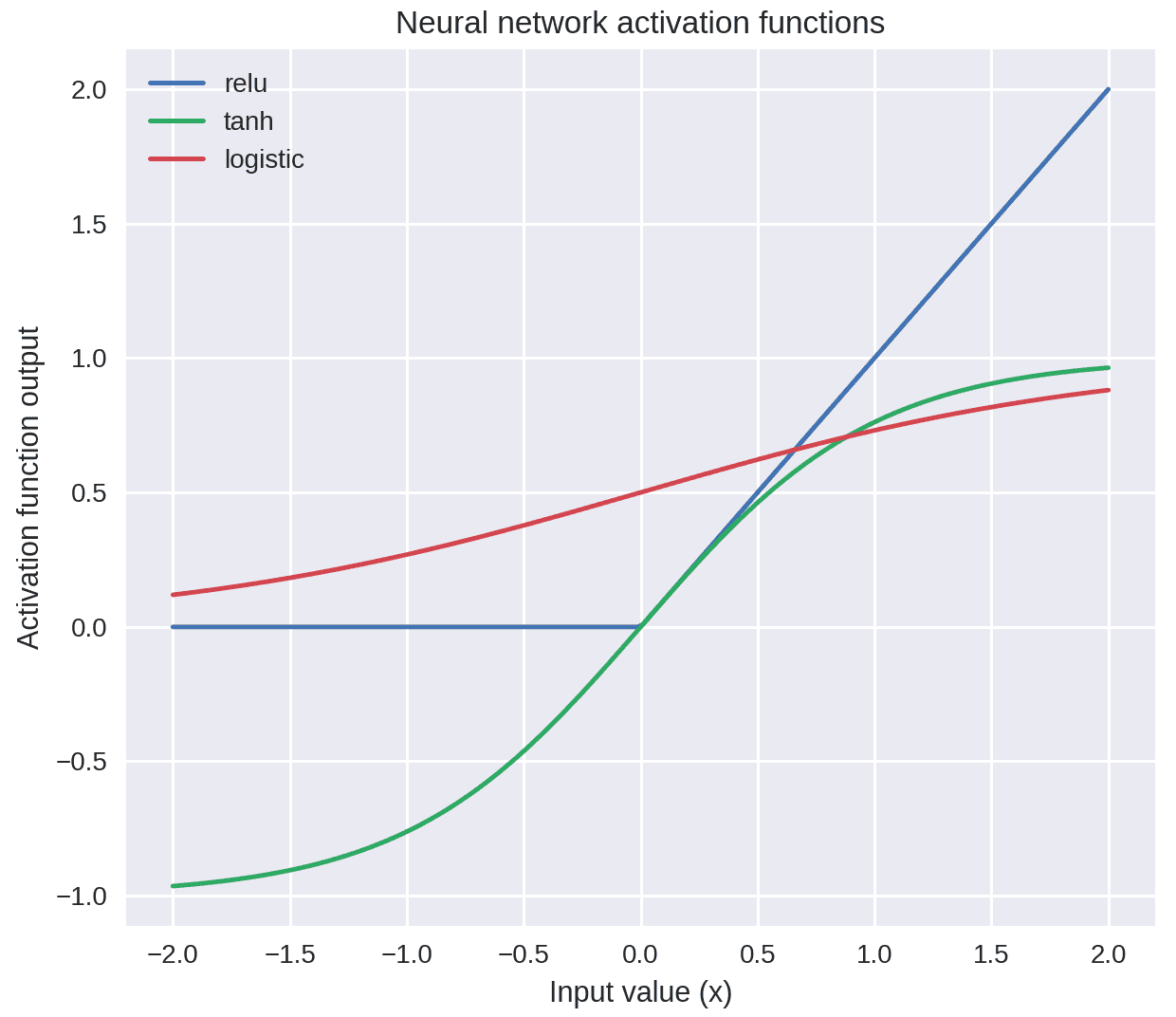## Application

### logistic

One hidden layer

from sklearn.neural_network import MLPClassifier

X_train, X_test, y_train, y_test = train_test_split(X_D2, y_D2, random_state=0)

fig, subaxes = plt.subplots(3, 1, figsize=(6,18))

for units, axis in zip([1, 10, 100], subaxes):
nnclf = MLPClassifier(hidden_layer_sizes = [units], solver='lbfgs',
random_state = 0).fit(X_train, y_train)

title = 'Dataset 1: Neural net classifier, 1 layer, {} units'.format(units)

plot_class_regions_for_classifier_subplot(nnclf, X_train, y_train,
X_test, y_test, title, axis)
plt.tight_layout()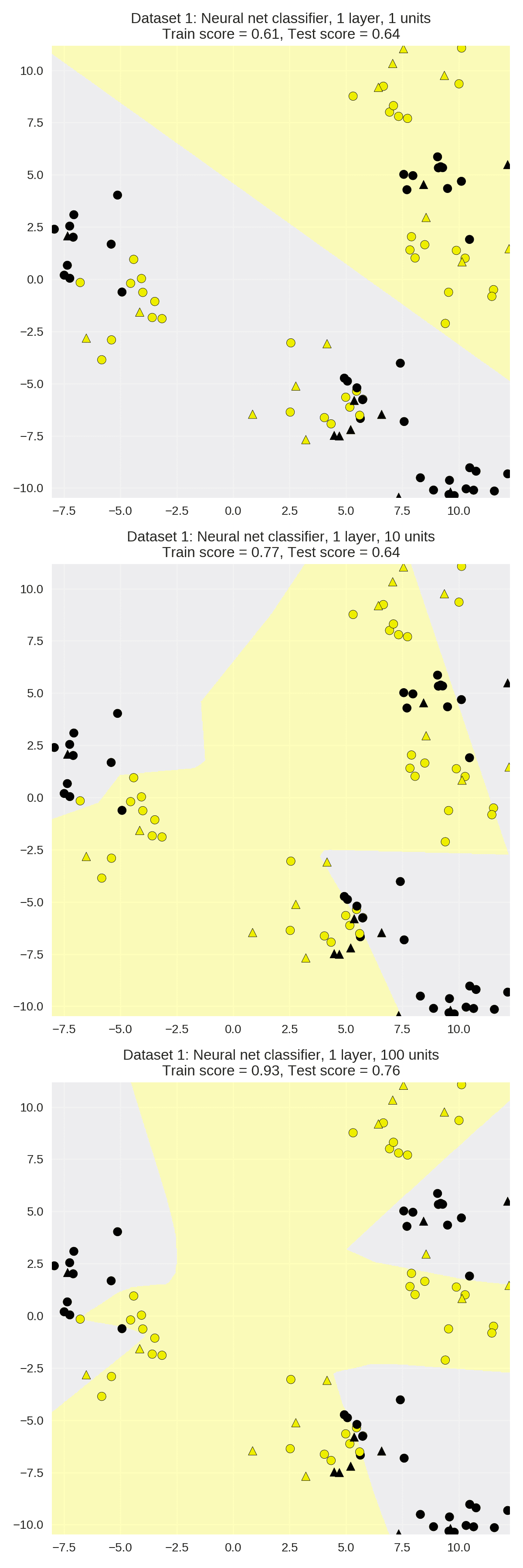Two hidden layers

from adspy_shared_utilities import plot_class_regions_for_classifier

X_train, X_test, y_train, y_test = train_test_split(X_D2, y_D2, random_state=0)

nnclf = MLPClassifier(hidden_layer_sizes = [10, 10], solver='lbfgs',
random_state = 0).fit(X_train, y_train)

plot_class_regions_for_classifier(nnclf, X_train, y_train, X_test, y_test,
'Dataset 1: Neural net classifier, 2 layers, 10/10 units')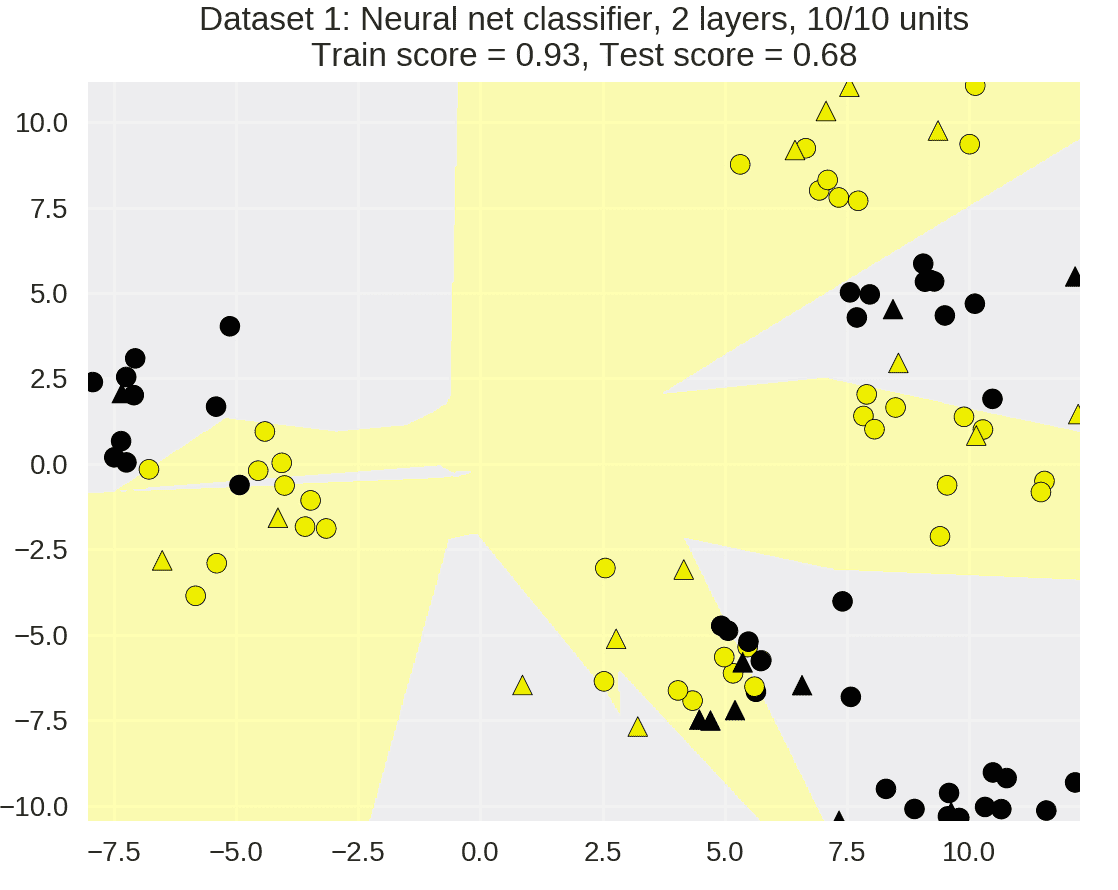### Regression(MLPRegressor)

from sklearn.neural_network import MLPRegressor

fig, subaxes = plt.subplots(2, 3, figsize=(11,8), dpi=70)

X_predict_input = np.linspace(-3, 3, 50).reshape(-1,1)

X_train, X_test, y_train, y_test = train_test_split(X_R1[0::5], y_R1[0::5], random_state = 0)

for thisaxisrow, thisactivation in zip(subaxes, ['tanh', 'relu']):
for thisalpha, thisaxis in zip([0.0001, 1.0, 100], thisaxisrow):
mlpreg = MLPRegressor(hidden_layer_sizes = [100,100],
activation = thisactivation,
alpha = thisalpha,
solver = 'lbfgs').fit(X_train, y_train)
y_predict_output = mlpreg.predict(X_predict_input)
thisaxis.set_xlim([-2.5, 0.75])
thisaxis.plot(X_predict_input, y_predict_output,
'^', markersize = 10)
thisaxis.plot(X_train, y_train, 'o')
thisaxis.set_xlabel('Input feature')
thisaxis.set_ylabel('Target value')
thisaxis.set_title('MLP regression\nalpha={}, activation={})'
.format(thisalpha, thisactivation))
plt.tight_layout()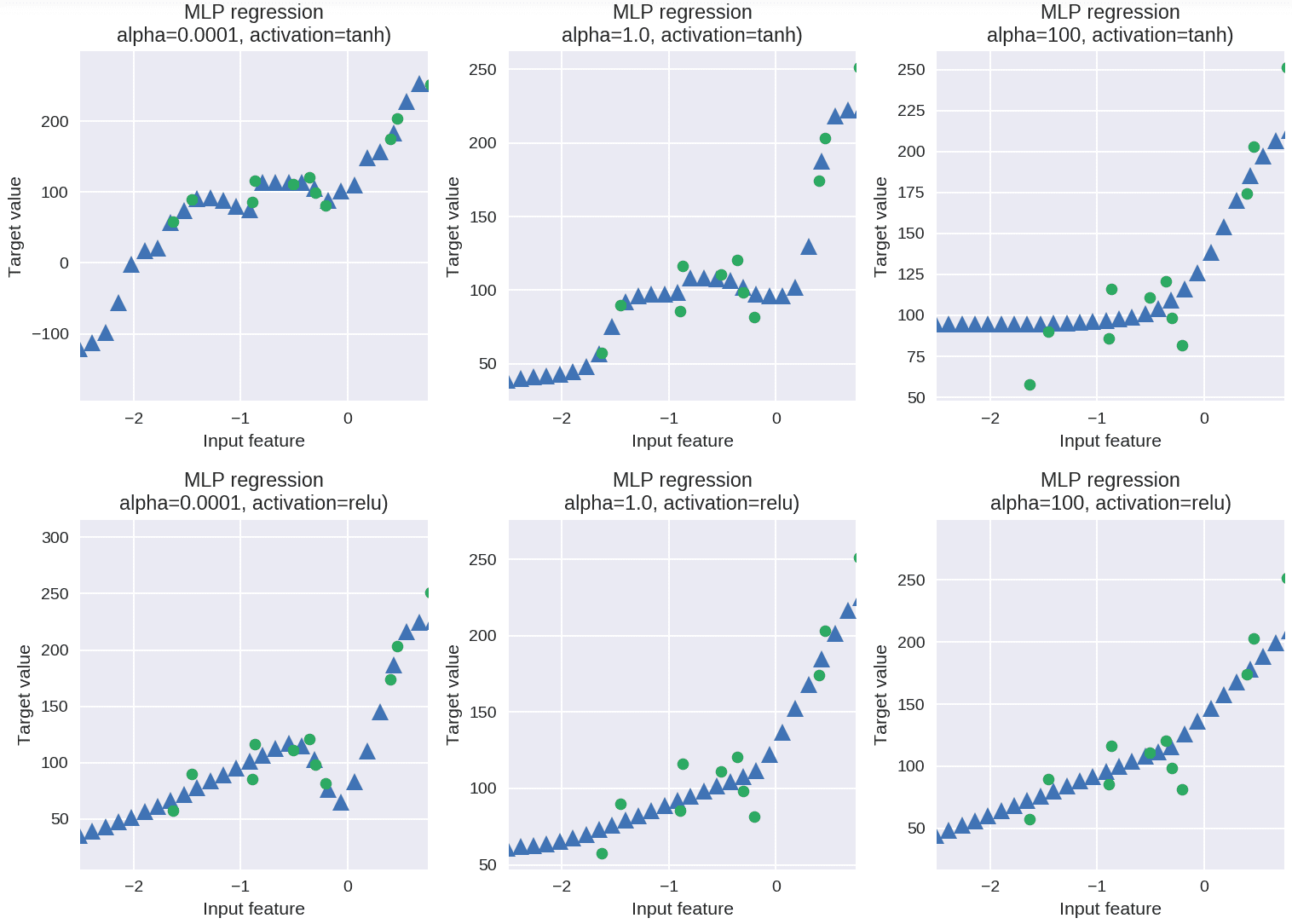## Pros and cons

L2 regularization with the alpha parameter

MLP Regressor

Data Leakage

Prediction target

• 588
• 0

### YOU MIGHT ALSO LIKE

0 0 vote
Article Rating
Subscribe0 评论
Inline Feedbacks